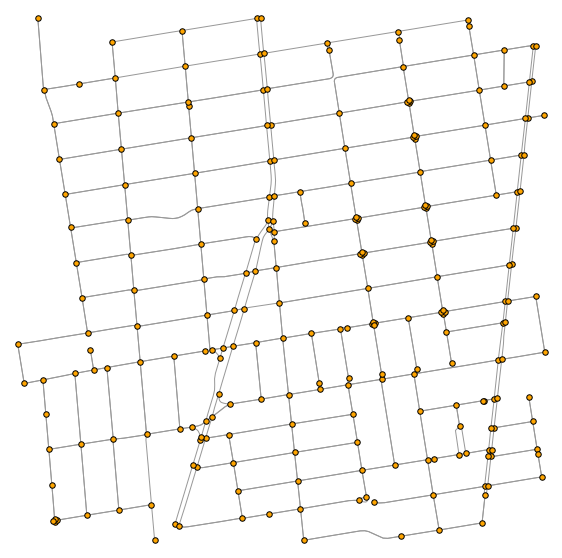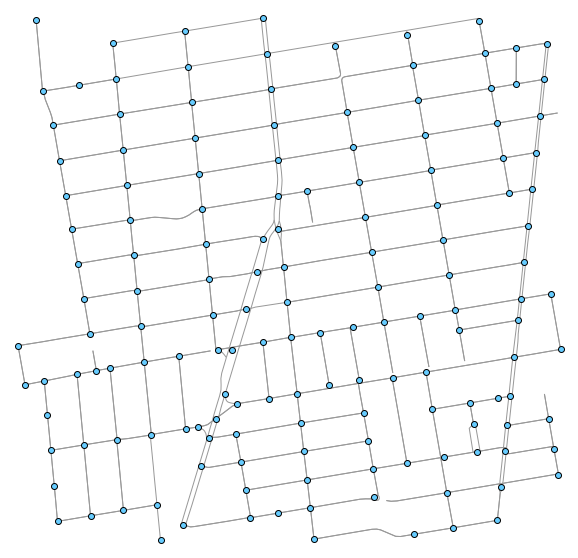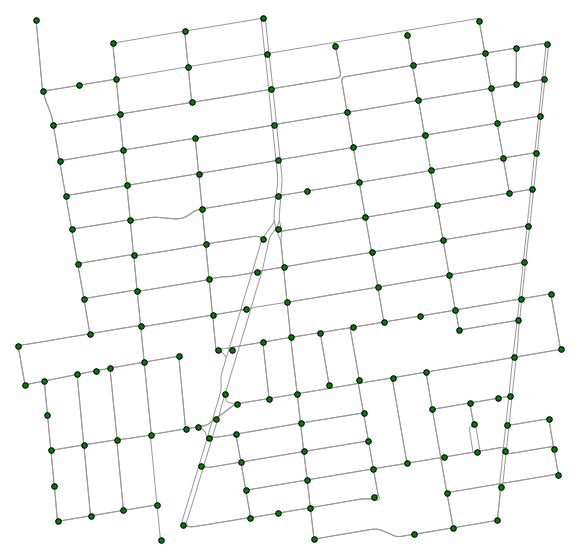### Introduction

It has been a while since I have posted anything on Python, so I thought it is time to switch things up and write do a Python GIS tutorial. GIS in python typically revolves around the geopandas and shapely packages. If you are using OpenStreetMaps(osm) in your work, the osmnx package is also very useful and makes downloading and visualising map data straightforward.

In this post, I explore the problem of simplifying route intersections. An intersection of two divided roads creates four nodes, at the points where an edge intersects another edge. While this level of detail is useful if one wants to know the possible turning options while driving down a road, often times this is not needed. Simplifying the map by aggregating the route intersection nodes into a single point makes modeling the map as a graph easier to comprehend and visualise.

The osmnx package has a clean_intersection function which returns the centroids of intersections. However, it does actually modify the graph which makes it not convenient for further analytical work. This post builds on the existing function and provides a solution to modify the road network and visualise it. I will use the example as this notebook so one can easily compare both approaches.

### Code

Let’s first import the required modules and replicate the example in the notebook.

import numpy as np
import pandas as pd
import osmnx as ox
import networkx as nx
from geopandas import gpd
from osmnx import graph_to_gdfs, gdfs_to_graph, save_and_show, get_paths_to_simplify
from shapely.geometry import Point, LineString, shape, MultiPoint, box, Polygon, MultiLineString, mapping
from shapely.ops import linemerge
import matplotlib.pyplot as plt
from matplotlib.collections import LineCollection

# get a street network and plot it with all edge intersections
address = '2700 Shattuck Ave, Berkeley, CA'

fig, ax = ox.plot_graph(G, fig_height=10, node_color='orange', node_size=30,
node_zorder=2, node_edgecolor='k')# clean up the intersections and extract their xy coords
G = ox.project_graph(G)
intersections = ox.clean_intersections(G, tolerance=15, dead_ends=False)
points = np.array([point.xy for point in intersections])

# plot the cleaned-up intersections
fig, ax = ox.plot_graph(G, fig_height=10, show=False, close=False, node_alpha=0)
ax.scatter(x=points[:,0], y=points[:,1], zorder=2, color='#66ccff',
edgecolors='k')
plt.show()Notice that the centroids are overlayed on the original graph with node_alpha set to zero. This is because osmnx’s clean_intersection function only returns the centroids but does not actually modify the underlying dataframe or graph structure. Let us take a look at the source code to understand it a little better.

### Clean intersections source code

# if dead_ends is False, discard dead-end nodes to only work with edge
# intersections
if 'streets_per_node' in G.graph:
streets_per_node = G.graph['streets_per_node']
else:
streets_per_node = count_streets_per_node(G)

dead_end_nodes = [node for node, count in streets_per_node.items()
if count <= 1]
G = G.copy()

# create a GeoDataFrame of nodes, buffer to passed-in distance, merge
# overlaps
gdf_nodes = graph_to_gdfs(G, edges=False)
buffered_nodes = gdf_nodes.buffer(tolerance).unary_union
if isinstance(buffered_nodes, Polygon):
# if only a single node results, make it iterable so we can turn it into
# a GeoSeries
buffered_nodes = [buffered_nodes]

# get the centroids of the merged intersection polygons
unified_intersections = gpd.GeoSeries(list(buffered_nodes))
intersection_centroids = unified_intersections.centroid
return intersection_centroids


It buffers the nodes, merges the ones that overlap and returns the centroid of the overlapping nodes. Instead of returning the node centroid, we want to get back a dataframe with the new nodes. This means that we will have to create a new node in the dataframe and replace the ones that were merge with it. In addition, the edges have to be modified as well to contain the information of the previous nodes within the identity of the new merged node.

### Modifying the function

First, let’s buffer the points and get the union of these buffered points:

gdf_nodes, gdf_edges = graph_to_gdfs(G)
buffered_nodes = gdf_nodes.buffer(15).unary_union
unified_intersections = gpd.GeoSeries(list(buffered_nodes))
unified_gdf = gpd.GeoDataFrame(unified_intersections).rename(columns={0:'geometry'}).set_geometry('geometry')
unified_gdf.crs = gdf_nodes.crs

geometry
0 POLYGON ((564163.0573396974 4189598.420167893,...
1 POLYGON ((564586.2116373704 4189601.780093063,...
2 POLYGON ((564861.6779513167 4189615.534235777,...
3 POLYGON ((565091.7608962897 4189655.09088124, ...
4 POLYGON ((564970.2509510979 4189633.912446446,...

Next, we merge the original nodes with the aggregated polygons. Geopandas has a sjoin function which uses rtrees for spatial joins, making it very efficient. We will just assign the smallest osmid to be the id of the new node.

# Merge original nodes with the aggregated shapes
intersections = gpd.sjoin(gdf_nodes, unified_gdf, how="right", op='intersects')
intersections['geometry_str'] = intersections['geometry'].map(lambda x: str(x))
intersections['new_osmid'] = intersections.groupby('geometry_str')['index_left'].transform('min').astype(str)
intersections['num_osmid_agg'] = intersections.groupby('geometry_str')['index_left'].transform('count')


The modifications to the vertices are almost complete. We will store the vertices which were affected by the union operation in a temporary lookup dataframe.

# Create temporary lookup with the agg osmid and the new one
lookup = intersections[intersections['num_osmid_agg']>1][['osmid', 'new_osmid', 'num_osmid_agg']]
lookup = lookup.rename(columns={'osmid': 'old_osmid'})
intersections = intersections[intersections['osmid'].astype(str)==intersections['new_osmid']]
intersections = intersections.set_index('index_left')

# Make everything else similar to original node df
intersections = intersections[gdf_nodes.columns]
intersections['geometry'] = intersections.geometry.centroid
intersections['x'] = intersections.geometry.x
intersections['y'] = intersections.geometry.y
del intersections.index.name
intersections.gdf_name = gdf_nodes.gdf_name


This brings us to the more tedious and complicated part - modifying the edges of the existing graph. It is not immediately clear how the edges has be modified and which geometries should be retained. I will get to managing the geometries slightly later. First, let’s consider how the set of edges could be affected by the union operation on the vertices. Since an edges is a u-v pair, where u and v are an ID of a vertex, there are three possibilities to consider:
1) Neither the start (u) or end (v) of the edge is affected by the union operation.
2) Either u or v is affected by the union.
3) Both u and v are affected by the union.

We merge the lookup table to the edge dataframe to identify these three cases.

agg_gdf_edges = pd.merge(gdf_edges.assign(u=gdf_edges.u.astype(str)),
lookup.rename(columns={'new_osmid': 'new_osmid_u',
'old_osmid': 'old_osmid_u'}),
left_on='u', right_on='old_osmid_u', how='left')
agg_gdf_edges = pd.merge(agg_gdf_edges.assign(v=agg_gdf_edges.v.astype(str)),
lookup.rename(columns={'new_osmid': 'new_osmid_v',
'old_osmid': 'old_osmid_v'}),
left_on='v', right_on='old_osmid_v', how='left')


Case 1 is straightforward and we can simply reuse the edges in their original form. For cases 2 and 3, we want to copy over the geometries to the chosen aggregated node. There is a slight complication if both ends of an edge are not in the set of retained vertices (i.e. they are both merged and mapped to another vertex ID). This will be quite common at road junctions where there are multiple divided roads. We still want to retain the look of the road (it’s geometry) while combining the vertices.

After experimenting with a few different approaches, my preferred method would be to create a self-loop to contain these geometries. The allows the map to look exactly the same as before and does not interfere with any shortest path solutions.

The code below implements the above logic. We make use of the very handy linemerge function in the shapely package to combine the different line segments.

# Remove all u-v edges that are between the nodes that are aggregated together (case 3)
agg_gdf_edges_c3 = agg_gdf_edges[((agg_gdf_edges['new_osmid_v'].notnull()) &
(agg_gdf_edges['new_osmid_u'].notnull()) &
(agg_gdf_edges['new_osmid_u'] == agg_gdf_edges['new_osmid_v']))]

agg_gdf_edges = agg_gdf_edges[~agg_gdf_edges.index.isin(agg_gdf_edges_c3.index)]

# Create a self loop containing all the joint geometries of the aggregated nodes where both u and v are agg
# Set onway to false to prevent duplication if someone were to create bidrectional edges
agg_gdf_edges_int = agg_gdf_edges_c3[
~((agg_gdf_edges_c3['new_osmid_u'] == agg_gdf_edges_c3['u']) |
(agg_gdf_edges_c3['new_osmid_v'] == agg_gdf_edges_c3['v']))
]
agg_gdf_edges_int = agg_gdf_edges_int.dissolve(by=['new_osmid_u', 'new_osmid_v']).reset_index()
agg_gdf_edges_int['u'] = agg_gdf_edges_int['new_osmid_u']
agg_gdf_edges_int['v'] = agg_gdf_edges_int['new_osmid_v']
agg_gdf_edges_int = agg_gdf_edges_int[gdf_edges.columns]
agg_gdf_edges_int['oneway'] = False

# Simplify by removing edges that do not involve the chosen agg point
# at least one of them must contain the new u or new v
agg_gdf_edges_c3 = agg_gdf_edges_c3[
(agg_gdf_edges_c3['new_osmid_u'] == agg_gdf_edges_c3['u']) |
(agg_gdf_edges_c3['new_osmid_v'] == agg_gdf_edges_c3['v'])
]

agg_gdf_edges_c3 = agg_gdf_edges_c3[['geometry', 'u', 'v', 'new_osmid_u',
'new_osmid_v']]
agg_gdf_edges_c3.columns = ['old_geometry', 'old_u', 'old_v', 'new_osmid_u',
'new_osmid_v']

# Merge back the linestring for case 2
# Ignore u and v if they are on the merging / agg node
# Copy over the linestring only on the old node
subset_gdf = agg_gdf_edges_c3[agg_gdf_edges_c3['new_osmid_v']!=
agg_gdf_edges_c3['old_v']]
agg_gdf_edges = pd.merge(agg_gdf_edges, subset_gdf[['old_geometry', 'old_v']],
how='left', left_on='u', right_on='old_v')

geom = agg_gdf_edges[['geometry', 'old_geometry']].values.tolist()
agg_gdf_edges['geometry'] = [linemerge([r, r]) if isinstance(r,
(LineString, MultiLineString)) else r for r in geom]
agg_gdf_edges.drop(['old_geometry', 'old_v'], axis=1, inplace=True)


Just a little more clean up and we are about done!

subset_gdf = agg_gdf_edges_c3[agg_gdf_edges_c3['new_osmid_u']!=
agg_gdf_edges_c3['old_u']]
agg_gdf_edges = pd.merge(agg_gdf_edges, subset_gdf[['old_geometry', 'old_u']],
how='left', left_on='v', right_on='old_u')

geom = agg_gdf_edges[['geometry', 'old_geometry']].values.tolist()
agg_gdf_edges['geometry'] = [linemerge([r, r]) if isinstance(r,
(LineString, MultiLineString)) else r for r in geom]
agg_gdf_edges.drop(['old_geometry', 'old_u'], axis=1, inplace=True)

agg_gdf_edges['u'] = np.where(agg_gdf_edges['new_osmid_u'].notnull(), agg_gdf_edges['new_osmid_u'], agg_gdf_edges['u'])
agg_gdf_edges['v'] = np.where(agg_gdf_edges['new_osmid_v'].notnull(), agg_gdf_edges['new_osmid_v'], agg_gdf_edges['v'])
agg_gdf_edges = agg_gdf_edges[gdf_edges.columns]
agg_gdf_edges = gpd.GeoDataFrame(pd.concat([agg_gdf_edges, agg_gdf_edges_int],
ignore_index=True), crs=agg_gdf_edges.crs)

agg_gdf_edges['u'] = agg_gdf_edges['u'].astype(np.int64)
agg_gdf_edges['v'] = agg_gdf_edges['v'].astype(np.int64)


I saved the entire code chunk as a function called clean_intersections_graph. Let’s give it a test run.

G2 = ox.graph_from_address(address, network_type='drive', distance=750)
G2 = ox.project_graph(G2)
G2_clean = clean_intersections_graph(G2, tolerance=15, dead_ends=False)
ox.plot_graph(G2_clean, node_color='green', node_size=30, node_zorder=2, node_edgecolor='k')

---------------------------------------------------------------------------

NotImplementedError                       Traceback (most recent call last)

<ipython-input-78-6d9db8af087d> in <module>
2 G2 = ox.project_graph(G2)
3 G2_clean = clean_intersections_graph(G2, tolerance=15, dead_ends=False)
----> 4 ox.plot_graph(G2_clean, node_color='green', node_size=30, node_zorder=2, node_edgecolor='k')

~\AppData\Local\Continuum\anaconda3\lib\site-packages\osmnx\plot.py in plot_graph(G, bbox, fig_height, fig_width, margin, axis_off, equal_aspect, bgcolor, show, save, close, file_format, filename, dpi, annotate, node_color, node_size, node_alpha, node_edgecolor, node_zorder, edge_color, edge_linewidth, edge_alpha, use_geom)
377             # if it has a geometry attribute (a list of line segments), add them
378             # to the list of lines to plot
--> 379             xs, ys = data['geometry'].xy
380             lines.append(list(zip(xs, ys)))
381         else:

~\AppData\Local\Continuum\anaconda3\lib\site-packages\shapely\geometry\base.py in xy(self)
333     def xy(self):
334         """Separate arrays of X and Y coordinate values"""
--> 335         raise NotImplementedError
336
337     # Python feature protocol

NotImplementedError:


### Handling linestrings and multilinestrings

We get a NotImplementedError when xs, ys = data['geometry'].xy is called. To understand what caused the problem, we have to take a look at the linemerge function in more detail. I created 3 line segments below and ran linemerge on two different pairs.

line0 = [[0, 0], [1, 1]]
line1 = [[1, 1], [2, 1]]
line2 = [[0.5, 0], [0.5, 2]]

line = linemerge([line0, line1])
print(line)
line

LINESTRING (0 0, 1 1, 2 1)line = linemerge([line0, line2])
print(line)
line

MULTILINESTRING ((0 0, 1 1), (0.5 0, 0.5 2))In the first case, where the line is continuous, linemerge returns a linestring. In the second case, where the merge object is not continuous, linemerge returns a multilinestring containing the line sequences. While a linestring object has a .xy method, multilinestring does not, hence, the NotImplementedError which we previously encountered. To solve this problem, we can use the mapping function to get the coordinates instead.

Create a plot_graph_mls function and replace the contents in the if 'geometry' in data and use_geom: clause, with the code below:

if isinstance(data['geometry'], MultiLineString):
lines += [list(t) for t in mapping(data['geometry'])['coordinates']]
else:
lines += [list(mapping(data['geometry'])['coordinates'])]


Let’s try it out once again with the new multilinestring plotting function. This time round we get the same map as in the original example from the osmnx package but also retain the aggregated nodes as a networkx graph object which could be used for other analytical projects.

### Test new plot function which allows MultiLineString to be plotted